# H.2 Recursive Algorithm to Calculate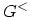Following Appendix H.1, the algorithm to calculate the electron density (diagonal elements of) is discussed in terms of the DYSON equation for the lesser and the left-connected GREEN's functions. The solution to the matrix equation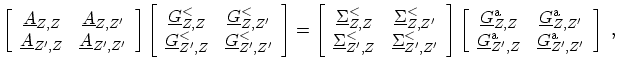(H.12)

can be written as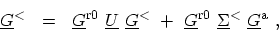(H.13)

where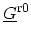and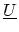have been defined in (H.7) and (H.8), and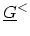and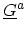are readily identifiable from (H.12). Using the relation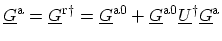, (H.13) can be written as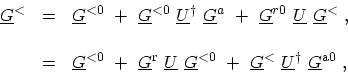(H.14)

where(H.15)

The left-connected lesser GREEN's function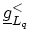is defined by the first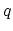blocks of (H.2)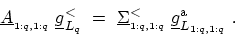(H.16)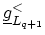is defined in a manner identical toexcept that the left-connected system is comprised of the first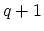blocks of (H.2). In terms of (H.12), the equation governingfollows by setting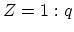and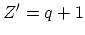. Using the DYSON equations for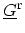and,can be recursively obtained as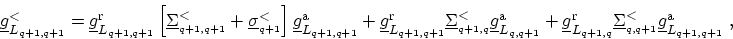(H.17)

which can be written in a more intuitive form as(H.18)

where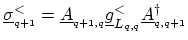. Equation (H.18) has the physical meaning that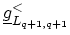has contributions due to four injection functions: (i) an effective self-energy due to the left-connected structure that ends at, which is represented by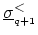, (ii) the diagonal self-energy component at grid pointthat enters (H.2), and (iii) the two off-diagonal self-energy components involving grid pointsand.

To express the full lesser GREEN's function in terms of the left-connected GREEN's function, one can consider (H.12) such that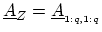,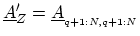and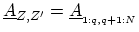. Noting that the only non-zero block of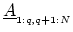isand using (H.14), one obtains(H.19)

Using (H.14),can be written in terms of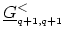and other known GREEN's functions as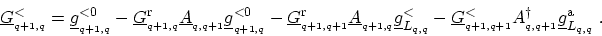(H.20)

Substituting (H.20) in (H.19) and using (H.5), one obtains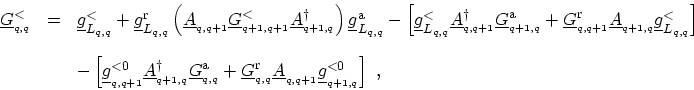(H.21)

where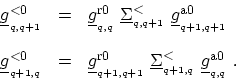(H.22)

The terms inside the square brackets of (H.21) are HERMITian conjugates of each other. In view of the above equations, the algorithm to compute the diagonal blocks ofis given by the following steps:
•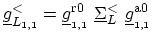,
• For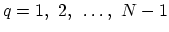, (H.18) is computed,
• For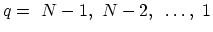, (H.21) and (H.22) are computed.

M. Pourfath: Numerical Study of Quantum Transport in Carbon Nanotube-Based Transistors